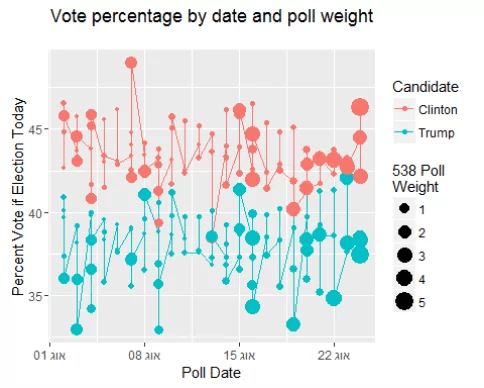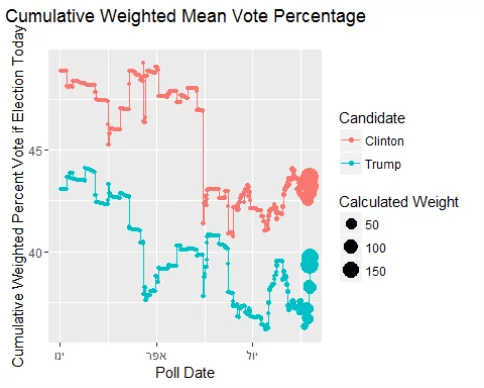Want to share your content on R-bloggers? click here if you have a blog, or here if you don't.

Guest post by Jo professor of mathematics, Pomona College.

ASA’s Prediction Competition

In this election year, the American Statistical Association (ASA) has put together a competition for students to predict the exact percentages for the winner of the 2016 presidential election. They are offering cash prizes for the entry that gets closest to the national vote percentage and that best predicts the winners for each state and the District of Columbia. For more details see:

http://thisisstatistics.org/electionprediction2016/

To get you started, I’ve written an analysis of data scraped from fivethirtyeight.com. The analysis uses weighted means and a formula for the standard error (SE) of a weighted mean. For your analysis, you might consider a similar analysis on the state data (what assumptions would you make for a new weight function?). Or you might try some kind of model – either a generalized linear model or a Bayesian analysis with an informed prior. The world is your oyster!

Getting the Data

Thanks to the Internet, there is a lot of polling data which is publicly accessible. For the competition, you are welcome to get your data from anywhere. However, I’m going to take mine from 538. http://projects.fivethirtyeight.com/2016-election-forecast/national-polls/ (Other good sources of data are http://www.realclearpolitics.com/epolls/latest_polls/ and http://elections.huffingtonpost.com/pollster/2016-general-election-trump-vs-clinton and http://www.gallup.com/products/170987/gallup-analytics.aspx)

Note the date indicated above as to when this R Markdown file was written. That’s the day the data were scraped from 538. If you run the Markdown file on a different day, you are likely to get different results as the polls are constantly being updated.

Because the original data were scraped as a JSON file, it gets pulled into R as a list of lists. The data wrangling used to convert it into a tidy format is available from the source code at https://github.com/hardin47/prediction2016/blob/master/predblog.Rmd.

url = “http://projects.fivethirtyeight.com/2016-election-forecast/national-polls/”
doc <- htmlParse(url, useInternalNodes = TRUE)

sc = xpathSApply(doc, “//script[contains(., ‘race.model’)]”,
function(x) c(xmlValue(x), xmlAttrs(x)[[“href”]]))

jsobj = gsub(“.*race.stateData = (.*);race.pathPrefix.*”, \\1″, sc)

data = fromJSON(jsobj)
allpolls <- data\$polls

#unlisting the whole thing
indx <- sapply(allpolls, length)
pollsdf <- as.data.frame(do.call(rbind, lapply(allpolls, `length<-`, max(indx))))

A Quick Visualization

Before coming up with a prediction for the vote percentages for the 2016 US Presidential Race, it is worth trying to look at the data. The data are in a tidy form, so ggplot2 will be the right tool for visualizing the data.

ggplot(subset(allpolldata, ((polltypeA == “now”) & (endDate > ymd(“2016-08-01”)))),
geom_line() + geom_point(aes(size=wtnow)) +
labs(title = “Vote percentage by date and poll weight\n,
y = “Percent Vote if Election Today”, x = “Poll Date”,
color = “Candidate”, size=“538 Poll\nWeight”)A Quick Analysis

Let’s try to think about the percentage of votes that each candidate will get based on the now cast polling percentages. We’d like to weight the votes based on what 538 thinks (hey, they’ve been doing this longer than I have!), the sample size, and the number of days since the poll closed.Using my weight, I’ll calculate a weighted average and a weighted SE for the predicted percent of votes. (The SE of the weighted variance is taken from Cochran (1977) and cited in Gatz and Smith (1995).) The weights can be used to calculate the average or the running average for the now cast polling percentages.

allpolldata2 <- allpolldata %>%
filter(wtnow > 0) %>%
filter(polltypeA == “now”) %>%
mutate(dayssince = as.numeric(today() – endDate)) %>%
mutate(wt = wtnow * sqrt(sampleSize) / dayssince) %>%
mutate(votewt = wt*pct) %>%
group_by(choice) %>%
arrange(choice, -dayssince) %>%
mutate(cum.mean.wt = cumsum(votewt) / cumsum(wt)) %>%
mutate(cum.mean = cummean(pct))

Plotting the Cumulative Mean / Weighted Mean

In tidy format, the data are ready to plot. Note that the cumulative mean is much smoother than the cumulative weighted mean because the weights are much heavier toward the later polls.

ggplot(subset(allpolldata2, ( endDate > ymd(“2016-01-01”))),
aes(y=cum.mean, x=endDate, color=choice)) +
geom_line() + geom_point(aes(size=wt)) +
labs(title = “Cumulative Mean Vote Percentage\n,
y = “Cumulative Percent Vote if Election Today”, x = “Poll Date”,
color = “Candidate”, size=“Calculated Weight”)ggplot(subset(allpolldata2, (endDate > ymd(“2016-01-01”))),
aes(y=cum.mean.wt, x=endDate, color=choice)) +
geom_line() + geom_point(aes(size=wt)) +
labs(title = “Cumulative Weighted Mean Vote Percentage\n,
y = “Cumulative Weighted Percent Vote if Election Today”, x = “Poll Date”,
color = “Candidate”, size=“Calculated Weight”)Additionally, the weighted average and the SE of the average (given by Cochran (1977)) can be computed for each candidate. Using the formula, we have our prediction of the final percentage of the popular vote for each major candidate!

pollsummary <- allpolldata2 %>%
select(choice, pct, wt, votewt, sampleSize, dayssince) %>%
group_by(choice) %>%
summarise(mean.vote = weighted.mean(pct, wt, na.rm=TRUE),
std.vote = sqrt(weighted.var.se(pct, wt, na.rm=TRUE)))

pollsummary

## # A tibble: 2 x 3
##    choice mean.vote  std.vote
##
## 1 Clinton  43.64687 0.5073492
## 2   Trump  39.32071 1.1792667

Prediction is very difficult, especially about the future. – Niels Bohr

Along with good data sources, you should also be able to find information about prediction and modeling. I’ve provided a few resources to get you started.

Andrew Gelman: http://andrewgelman.com/2016/08/17/29654/

Christensen and Florence: http://www.amstat.org/misc/tasarticle.pdf and https://tofu.byu.edu/electionpollproject/